# 入门 | Tensorflow实战讲解神经网络搭建详细过程## 编辑 | 磐石

【磐创AI导读】：本文详细介绍了神经网络在实战过程中的构建与调节方式。主欢迎大家点击上方蓝字关注我们的公众号：磐创AI点击公众号下方文章精选系列文章了解更多。

## 之前我们讲了神经网络的起源、单层神经网络、多层神经网络的搭建过程、搭建时要注意到的具体问题、以及解决这些问题的具体方法。本文将通过一个经典的案例：MNIST手写数字识别，以代码的形式来为大家梳理一遍神经网络的整个过程。

### 一 、MNIST手写数字数据集介绍

MNIST手写数字数据集来源于是美国国家标准与技术研究所，是著名的公开数据集之一，通常这个数据集都会被作为深度学习的入门案例。数据集中的数字图片是由250个不同职业的人纯手写绘制，数据集获取的网址为：http://yann.lecun.com/exdb/mnist/。（下载后需解压）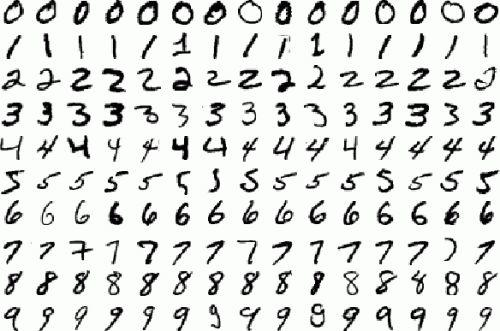### 二 、网络结构的设计

import tensorflow as tf

from tensorflow.examples.tutorials.mnist import input_data

batch_size=100

batch_num=mnist_data.train.num_examples//batch_sizex = tf.placeholder(tf.float32,[None,784])

y = tf.placeholder(tf.float32,[None,10])

weights = {

‘hidden_1’: tf.Variable(tf.random_normal([784, 256])),

‘out’: tf.Variable(tf.random_normal([256, 10]))

}

biases = {

‘b1’: tf.Variable(tf.random_normal()),

‘out’: tf.Variable(tf.random_normal())

}

def neural_network(x):

out_layer = tf.matmul(hidden_layer_1, weights[‘out’]) + biases[‘out’]

return out_layer

#调用神经网络

result = neural_network(x)

#预测类别

prediction = tf.nn.softmax(result)

#平方差损失函数

loss = tf.reduce_mean(tf.square(y-prediction))

#梯度下降法

#预测类标

correct_pred = tf.equal(tf.argmax(y,1),tf.argmax(prediction,1))

#计算准确率

accuracy = tf.reduce_mean(tf.cast(correct_pred,tf.float32))

#初始化变量

init = tf.global_variables_initializer()

step_num=400

with tf.Session() as sess:

sess.run(init)

for step in range(step_num+1):

for batch in range(batch_num):

batch_x,batch_y =  mnist_data.train.next_batch(batch_size)

sess.run(train_step,feed_dict={x:batch_x,y:batch_y})

acc = sess.run(accuracy,feed_dict={x:mnist_data.test.images,y:mnist_data.test.labels})

print(“Step ” + str(step) + “,Training Accuracy “+  “{:.3f}” + str(acc))

print(“Finished!”)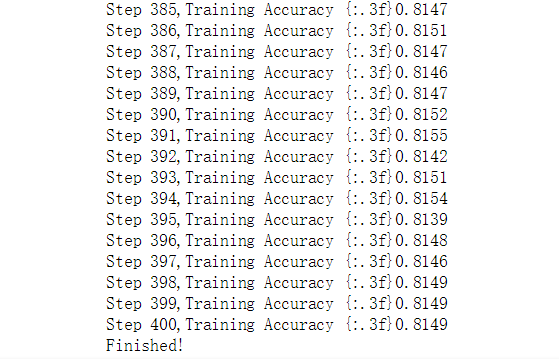#预测类别

prediction = tf.nn.softmax(result)

#交叉熵损失函数

loss = tf.reduce_mean(tf.nn.softmax_cross_entropy_with_logits(labels=y,logits=prediction))

#梯度下降法

#预测类标

correct_pred = tf.equal(tf.argmax(y,1),tf.argmax(prediction,1))

#计算准确率

accuracy = tf.reduce_mean(tf.cast(correct_pred,tf.float32))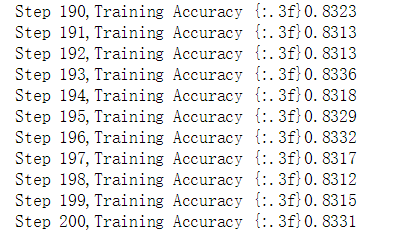Dropout是在每次神经网络的训练过程中，使得部分神经元工作而另外一部分神经元不工作。而测试的时候激活所有神经元，用所有的神经元进行测试。这样便可以有效的缓解过拟合，提高模型的准确率。具体代码如下所示：

def neural_network(x):

L1 = tf.nn.tanh(hidden_layer_1)

dropout1 = tf.nn.dropout(L1,0.5)

out_layer = tf.matmul(dropout1, weights[‘out’]) + biases[‘out’]

return out_layer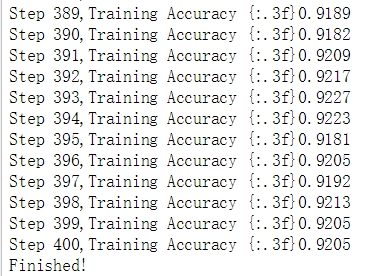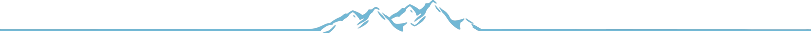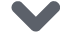点击下方 |  | 了解更多